# How to Find the Molar Mass

Molar mass is an important physical property of substances. It is very useful in analyzing, comparing and predicting the other physical and chemical properties such as density, melting point, boiling point, and the amount of substance that react with another substance in a system. In this article, we will be discussing five different methods; using atomic masses, equation, boiling point elevation, freezing point depression, and osmotic pressure, to calculate the molar mass.

### Key Areas Covered

1. What is Molar Mass
– Definition, Equation for Calculation, Explanation
2. How to Find the Molar Mass
– Several Methods to Calculate the Molar Mass with Examples
3. Why is it Important to Know the Molar Mass of a Substance
– Applications of Molar Mass

Key Terms: Avogadro’s Number, Boiling Point Elevation, Calusius-Clapeyron, Cryoscopic Constant, Ebullioscopic Constant, Freezing Point Depression, Molality, Molar Mass, Molecular Weight, Osmotic Pressure, Relative Atomic Mass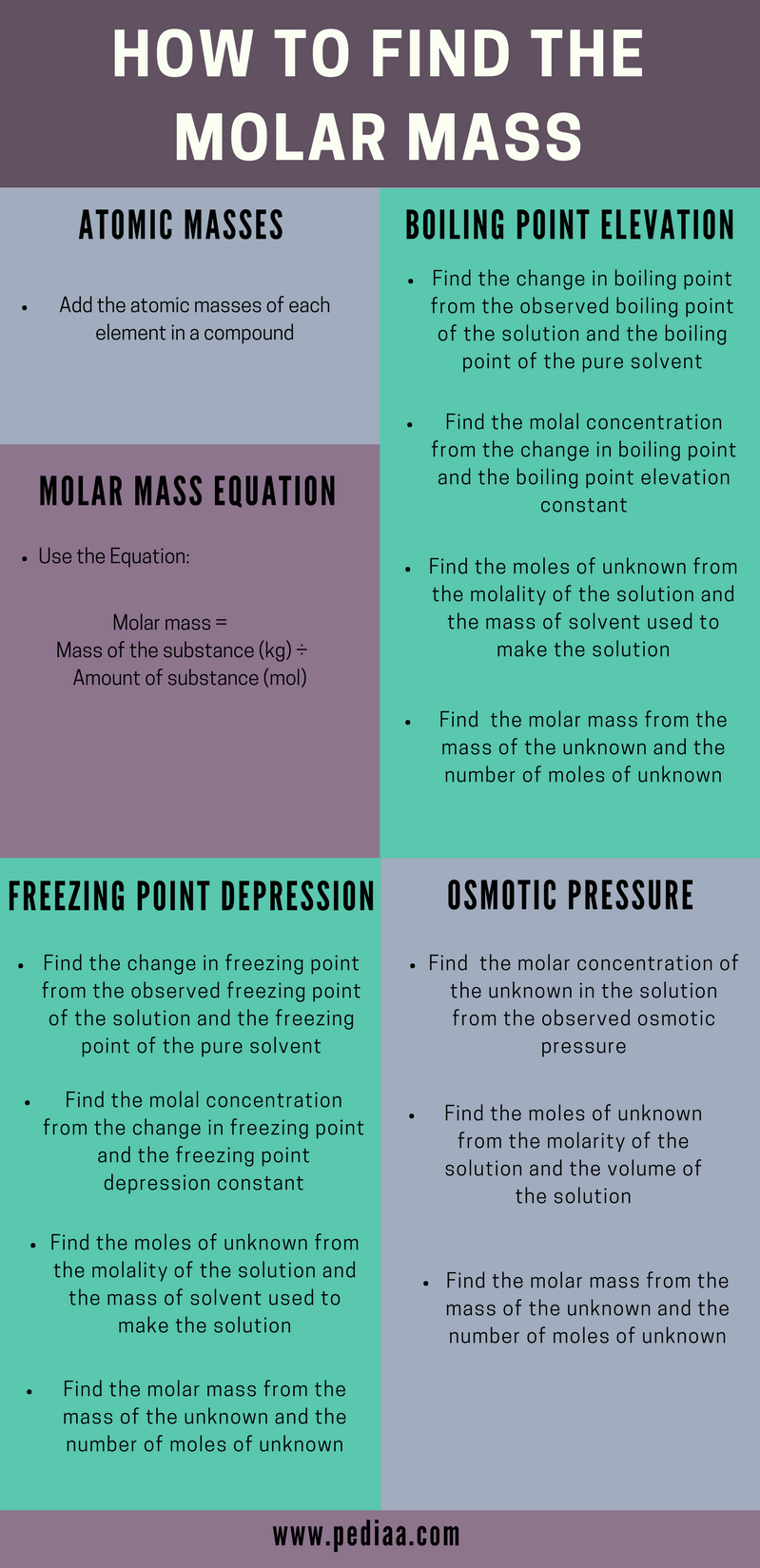## What is Molar Mass

Molar mass is the mass of a mole of a particular substance. The most commonly used unit for the molar mass of a substance is gmol-1. However, the SI unit for molar mass is kgmol-1 (or kg/mol). The molar mass can be calculated using the following equation.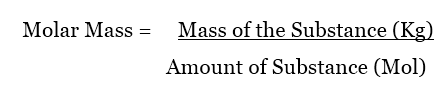Mole or mol is the unit used to measure the amount of a substance. One mole of a substance is equal to a very large number, 6.023 x 1023 of atoms (or molecules) which the substance is made of. This number is called the Avogadro’s number. It is a constant because no matter what the type of atom is, one mole of it is equal to that amount of atoms (or molecules). Therefore, the molar mass can be given a new definition, that is, molar mass is the total mass of 6.023 x 1023 atoms (or molecules) of a particular substance. To avoid confusion, take a look at the following example.

• Compound A is composed of A molecules.
• Compound B is composed of B molecules.
• One mole of compound A is composed of 6.023 x 1023 of A molecules.
• One mole of compound B is composed of 6.023 x 1023 of B molecules.
• Molar mass of compound A is the sum of masses of 6.023 x 1023 A molecules.
• Molar mass of compound B is the sum of masses of 6.023 x 1023 B molecules.

Now we can apply this for real substances. One mole of H2O is composed of 6.023 x 1023 H2O molecules. The total mass of 6.023 x 1023 H2O molecules is about 18 g. Therefore, the molar mass of H2O is 18 g/mol.

## How to Find the Molar Mass

The molar mass of a substance can be calculated using several methods.

These methods are discussed in detail below.

### 1. How to Find the Molar Mass Using Atomic Masses

The molar mass of a molecule can be determined using atomic masses. This can be done simply by the addition of molar masses of each atom present. Molar mass of an element is given as below.Relative atomic mass is the mass of an atom relative to the mass of Carbon-12 atom and it has no units. This relationship can be given as follows.

####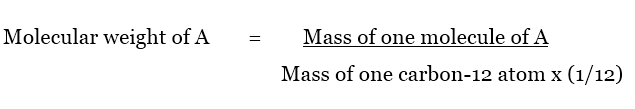Let’s consider following examples to understand this technique. Followings are the calculations for compounds with the same atom, combination of several different atoms and combination of a large number of atoms.

#### Examples of Calculation

##### 3. Molar Mass of C6H12O6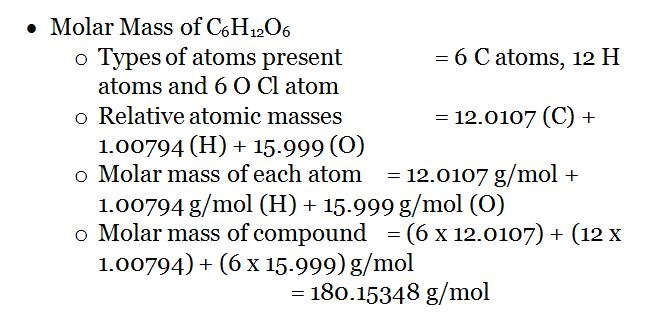### 2. How to Find the Molar Mass Using the Equation

The molar mass can be calculated using the equation given below. This equation is used to determine an unknown compound.

#### Example of Calculation

Take the following example.

The compound D is in a solution. The details are given as follows.

• Compound D is a strong base.
• It can release one H+ ion per molecule.
• The solution of compound D was made using 0.599 g of compound D.
• It reacts with HCl in the ratio of 1: 1

Then the determination can be done by an acid-base titration. Since it is a strong base, titrate the solution with a strong acid (Ex: HCl, 1.0 mol/L) in the presence of phenolphthalein indicator. The color change indicates the end point (Ex: when 15.00mL of HCl is added) of the titration and now all the molecules of the unknown base are titrated with the added acid. Then the molar mass of the unknown compound can be determined as follows.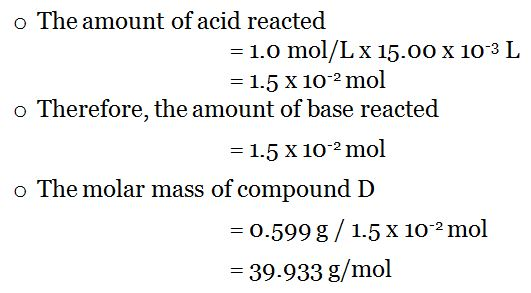### 3. How to Find the Molar Mass from Boiling Point Elevation

Boiling point elevation is the phenomena which describes that the addition of a compound to a pure solvent would increase the boiling point of that mixture to a higher boiling point than that of the pure solvent. Therefore, the molar mass of that added compound can be found using the temperature difference between two boiling points. If the boiling point of the pure solvent is Tsolvent and the boiling point of the solution (with the added compound) is Tsolution, the difference between two boiling points can be given as below.

####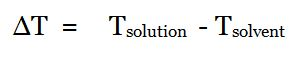With the use of Clausius-Clapeyron relation and Raoult’s law, we can get a relationship between ΔT and molality of the solution as,

####Where, Kb is ebullioscopic constant, which depends only on the properties of the solvent, and M is the molality.

From the above equation, we can get a value for molality of the solution. As the amount of solvent used for the preparation of this solution is known, we can find the value for moles of the compound added.

####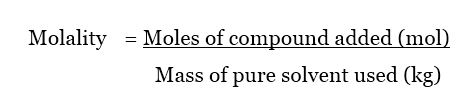Now that we know the moles of compound in the solution and the mass of the compound added, we can determine the molar mass of the compound using the equation,

### 4. How to Find the Molar Mass from Freezing Point Depression

Freezing point depression is the opposite of boiling point elevation. Sometimes, when a compound is added to a solvent, the freezing point of the solution is lowered than that of the pure solvent. Then the equations above are a little modified.

####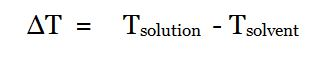The ΔT value is a minus value because the boiling point is now lower than the initial value. The molality of the solution can be obtained in the same way as in boiling point elevation method.

####Here, the Kf  is known as the cryoscopic constant. It is dependent only on the properties of the solvent.

The rest of the calculations are the same as in boiling point elevation method. Here, the moles of the compound added can also be calculated using the below equation.

####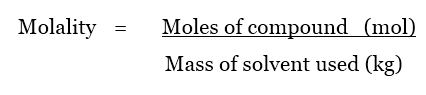Then the molar mass can be calculated using the value for moles of compound added and the mass of compound added.

### 5. How to Find the Molar Mass from Osmotic Pressure

Osmotic pressure is the pressure needed to be applied to avoid a pure solvent from passing to a given solution by osmosis. The osmotic pressure can be given by the following equation.

#### Π = MRT

When Π is the osmotic pressure,

M is the molarity of the solution

R is the universal gas constant

T is the temperature

The molarity of the solution is given by the following equation.

####The volume of the solution can be measured and the molarity can be calculated as above. Therefore, the moles of the compound in the solution can be measured. Then the molar mass can be determined using the equation,

## Why is it Important to Know the Molar Mass of a Substance

• Molar masses of different compounds can be used to compare the melting points and boiling points of those compounds.
• Molar mass is used to determine the mass percentages of atoms present in a compound.
• Molar mass is very important in chemical reactions to find out the amounts of a certain reactant that has reacted or to find the amount of the product that can be obtained.
• Knowing the molar masses is very important before an experimental set up is designed.

### Summary

There are several methods to calculate the molar mass of a given compound. The easiest way among them is the addition of molar masses of elements present in that compound.